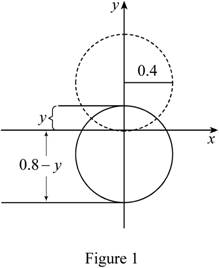# To show that: The percentage of the volume of the object above the surface of the liquid is ρ f − ρ o ρ f × 100### Single Variable Calculus: Concepts...

4th Edition
James Stewart
Publisher: Cengage Learning
ISBN: 9781337687805### Single Variable Calculus: Concepts...

4th Edition
James Stewart
Publisher: Cengage Learning
ISBN: 9781337687805

#### Solutions

Chapter 6, Problem 4P

(a)

To determine

## To show that: The percentage of the volume of the object above the surface of the liquid is ρf−ρoρf×100

Expert Solution

### Explanation of Solution

Given information:

The buoyant force is F=ρfgh0A(y)dy.

The weight of the object is W=ρoghLhA(y)dy.

Calculation:

Consider the volume above the surface as follows:

0LhA(y)dy (1)

Modify Equation (1).

0LhA(y)dy=hLhA(y)dyh0A(y)dy

Divide both sides of the Equation by hLhA(y)dy as shown below.

0LhA(y)dyhLhA(y)dy=hLhA(y)dyh0A(y)dyhLhA(y)dy (2)

Apply Archimedes Principle as shown below.

F=W

Substitute ρfgh0A(y)dy for F and ρoghLhA(y)dy for W in the above Equation.

ρfgh0A(y)dy=ρoghLhA(y)dyρfh0A(y)dy=ρohLhA(y)dyh0A(y)dy=ρoρfhLhA(y)dy

Substitute ρoρfhLhA(y)dy for h0A(y)dy in Equation (2).

0LhA(y)dyhLhA(y)dy=hLhA(y)dyρoρfhLhA(y)dyhLhA(y)dy=hLhA(y)dy(1ρoρf)hLhA(y)dy=1ρoρf=ρfρoρf

Find the percentage of the volume above the surface.

Percentage=ρfρoρf×100

Therefore, the percentage of the volume of the object above the surface of the liquid is ρfρoρf×100_ is proved.

(b)

To determine

### To calculate: The percentage of the volume of an iceberg above the water.

Expert Solution

The percentage of the volume of an iceberg above the water is 11%_.

### Explanation of Solution

Given information:

The density of ice is 917kg/m3.

The density of seawater is 1,030kg/m3.

Calculation:

Refer to part (a).

The percentage of the volume of the object above the surface of the liquid is Percentage=ρfρoρf×100.

Substitute 917kg/m3 for ρo and 1030kg/m3 for ρf in the above Equation.

Percentage=1,0309171,030×100=0.1097×100=10.97%=11%

Therefore, the percentage of the volume of an iceberg above the water is 11%_.

(c)

To determine

### To show: Does the water overflow when the ice melts?

Expert Solution

The water does not overflow when the ice melts.

### Explanation of Solution

Given information:

An ice cube floats in a glass filled to the brim with water.

Calculation:

Let Vi be the volume of the ice and Vw be the volume of the water from melting of ice.

Refer to part (a).

The volume of ice above the surface of the water is ρfρoρfVi.

The volume below the surface of the water is,

ViρfρoρfVi=ViρfViρf+Viρoρf=ρoρfVi

Suppose the mass of the ice cube is the same as the mass of the water which is formed when the cube melts.

ρoVi=ρfVwVw=ρoρfVi

So, when the ice cube melts the volume of the resulting water is same as the underwater volume of the ice cube.

Hence, the water does not overflow when the ice melts.

(d)

To determine

### To calculate: The work required to completely submerge the sphere.

Expert Solution

The work required to completely submerge the sphere is 1.05×103J_.

### Explanation of Solution

Given information:

A sphere of radius 0.4 m.

Density of the water is 1,000kg/m3.

Calculation:

Suppose the height of the exposed part of the ball is y.

Sketch the instant when the height of the exposed part of the ball is y as shown in Figure 1.Refer to Figure 1.

h=0.8y

Find the volume of the segment of a sphere as shown below.

V=13πh2(3rh) (3)

Substitute 0.4 m for r and (0.8y) for h in Equation (3).

V=13π×(0.8y)2(3×0.4(0.8y))=13π×(0.8y)2(1.2(0.8y))

Modify the above Equation as shown below.

V=13π×s2(1.2s)=13π(1.2s2s3)

Find the work done to submerge the sphere.

W=00.81000×9.81×13π(1.2s2s3)ds=10,273×(1.2s33s44)00.8=10,273×(1.2×0.8330.8440)=10,273×0.1024

=1,051.96J=1.05×103J

Therefore, the work required to completely submerge the sphere is 1.05×103J_.

### Have a homework question?

Subscribe to bartleby learn! Ask subject matter experts 30 homework questions each month. Plus, you’ll have access to millions of step-by-step textbook answers!